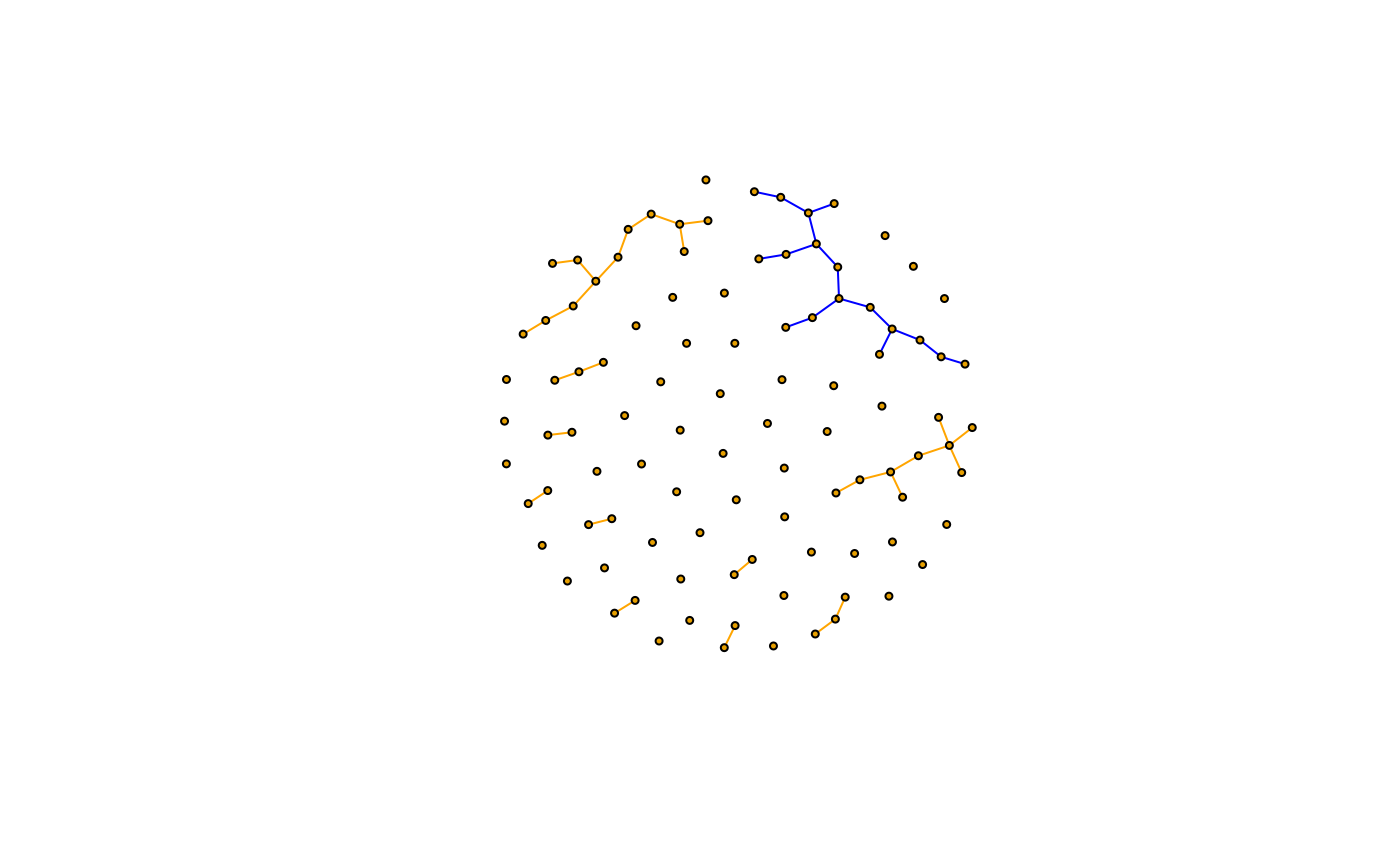$(x, name) <- value E(x, path = NULL, P = NULL, directed = NULL) <- value ## Arguments x An edge sequence. For E<- it is a graph. i Index. value New value of the attribute, for the edges in the edge sequence. name Name of the edge attribute to query or set. path Select edges along a path, given by a vertex sequence See E(). P Select edges via pairs of vertices. See E(). directed Whether to use edge directions for the path or P arguments. ## Value A vector or list, containing the values of the attribute name for the edges in the sequence. For numeric, character or logical attributes, it is a vector of the appropriate type, otherwise it is a list. ## Details The query form of $ is a shortcut for edge_attr(), e.g. E(g)[idx]$attr is equivalent to edge_attr(g, attr, E(g)[idx]). The assignment form of $ is a shortcut for set_edge_attr(), e.g. E(g)[idx]$attr <- value is equivalent to g <- set_edge_attr(g, attr, E(g)[idx], value). ## See also Other vertex and edge sequences: E(), V(), as_ids(), igraph-es-indexing2, igraph-es-indexing, igraph-vs-attributes, igraph-vs-indexing2, igraph-vs-indexing, print.igraph.es(), print.igraph.vs() Other vertex and edge sequences: E(), V(), as_ids(), igraph-es-indexing2, igraph-es-indexing, igraph-vs-attributes, igraph-vs-indexing2, igraph-vs-indexing, print.igraph.es(), print.igraph.vs() ## Examples # color edges of the largest component largest_comp <- function(graph) { cl <- components(graph) V(graph)[which.max(cl$csize) == cl$membership] } g <- sample_( gnp(100, 1 / 100), with_vertex_(size = 3, label = ""), with_graph_(layout = layout_with_fr) ) giant_v <- largest_comp(g) E(g)$color <- "orange"